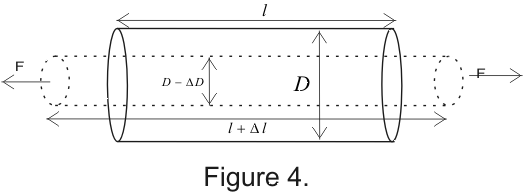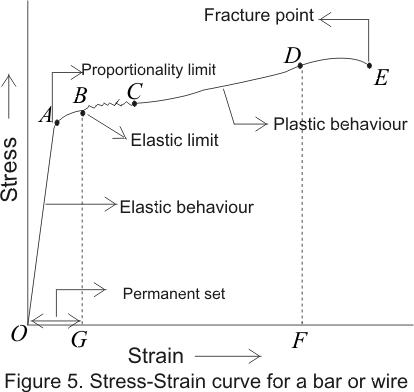# Poisson's Ratio and Stress Strain diagram

### 6. Poisson's Ratio

• When two equal and opposite forces are applied to a body in a certain direction , the body extends along that direction and at the same time it contracts along the perpendicular direction.
• The fractional change in length of the body in the direction of the applied forces is longitudinal strain and fractional change in the perpendicular direction of the force applied is called lateral strain.
• The ratio of lateral strain to the longitudinal strain is called Poisson's ratio which is constant for material of that body.
•• So when a body is subjected to strain , say an elongation, it also suffers contraction in perpendicularity direction.
• Within elastic limits, lateral strain β is is proportional to longitudinal strain α.hence
$\sigma=\frac{\beta}{\alpha}$
since,
longitudinal strain$= \alpha = \frac{\Delta l}{l}$
and
lateral strain$=\beta=\frac{\Delta D}{D}$
hence Poisson's ratio is
$\sigma=\frac{l\Delta D}{D\Delta L}$

### 7. Stress-Strain Digram

• In case of solids if we go on increasing stress continually then a point is reached at which strain increases more and more rapidly and Hook's law is no longer obeyed.
• Thus, the stress at which linear relationship between stress and strain ceases to hold is referred as elastic limit of material for the stress applied.
• If the elastic limit of material is exceeded it will fail to recover its original shape or size on removal of stress and would acquire a permanent set.
• Any type of stress can be plotted against appropriate strain and the shape of resulting stress- strain digrams would have shapes, depending on the kind of material.
• Simple stress- strain digram for a bar or wire is shown below in the figure.• (i). Portion OA is the straight line which clearly shows that stress produced is directly proportional to strain i.e., Hook's law is perfectly obeyed upto A and on removal of stress wire or bar will recover its original condition. Point A is called Proportionality limit

(ii). As soon as proportionality limit is crossed beyond point A, the strain increases more rapidly than stress and curve AB in graph shows that extension of wire in this limit is partly elastic and partly plastic and point B is the elastic limit of the material. Thus if we start decreasing load from point B the graph does not come to O via path BAO instead it traces straight line BG. So that there remains a residual strain. This is called permanent set.

(iii). If we continue to increases the stress beyond point B then for little or no increase in stress the strain increases rapidly up to point C.

(iv). Further increase of stress beyond point C produces a large increase in strain until a point E is reached at which fracture takes place and from B to D material is said to undergo plastic flow which is irreversible.
Conclusion :
1. The wire exhibits elasticity from O to b and plasticity from b to d. If the distance between b and d is more, then the metal is ductile. If the distance between b and d is small, then metal is brittle.
2. The substances which break as soon as the stress is increased beyond elastic limit are called brittle substances For example glass, cast iron, high carbon steel.
3. The substances which have a large plastic range are called ductile substances. For example copper, lead, gold, silver, iron, aluminum. Ductile materials can be drawn into wires. Malleable materials can be hammered into thin sheets. For example gold, silver, lead.